International
Tables for
Crystallography
Volume C
Mathematical, physical and chemical tables
Edited by E. Prince

International Tables for Crystallography (2006). Vol. C, ch. 8.7, p. 725

Section 8.7.3.9. Uncertainties in derived functions

P. Coppens,a Z. Sub and P. J. Beckerc

a732 NSM Building, Department of Chemistry, State University of New York at Buffalo, Buffalo, NY 14260-3000, USA,bDigital Equipment Co., 129 Parker Street, PKO1/C22, Maynard, MA 01754-2122, USA, and cEcole Centrale Paris, Centre de Recherche, Grand Voie des Vignes, F-92295 Châtenay Malabry CEDEX, France

8.7.3.9. Uncertainties in derived functions

| top | pdf |

The electrostatic moments are functions of the scale factor, the positional parameters x, y and z of the atoms, and their charge-density parameters κ and. The standard uncertainties in the derived moments are therefore dependent on the variances and covariances of these parameters.

If Mp represents the m × m variance–covariance matrix of the parameters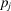, and T is an n × m matrix defined by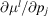for the lth moment with n independent elements, the variances and covariances of the elements of ml are obtained from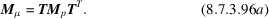If a moment of an assembly of pseudo-atoms is evaluated, the elements of T include the effects of coordinate rotations required to transfer atomic moments into a common coordinate system.

A frequently occurring case of interest is the evaluation of the magnitude of a molecular dipole moment and its standard deviation. Defining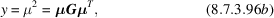whereis the dipole-moment vector and G is the direct-space metric tensor of the appropriate coordinate system. If Y is the 1 × 3 matrix of the derivatives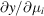,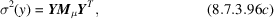where Mμ is defined by (8.7.3.96a). The standard uncertainty in μ may be obtained from σ(μ) = σ(y)/2μ. Significant contributions often result from uncertainties in the positional and charge-density parameters of the H atoms.